# 开发者实战 | AI分割一切——用OpenVINO™加速Meta SAM大模型

04/28 08:00

ChatGPT的火爆让大家看到了通用AI大模型的威力，也带动了近期一批自然语言处理（NLP）领域大模型的不断被推出。你方唱罢我登场，最近，计算机视觉领域也迎来了自己的物体分割大模型，由Meta开源的 “万物可分割 （Segment Anything Model, SAM）”物体分割模型

SAM是这两种方法的泛化，它是一个单一的模型，可以轻松地执行交互式分割和自动分割。SAM可以从输入提示（例如点或框）生成高质量的对象掩码，并且可以用于生成图像中所有对象的掩码。它已经在一个包含1.1亿个掩码的1100万个图像数据集上进行了训练，并且在各种分割任务上具有较强的零样本性能。它创建了一个通用的物体分割模型，可以在从分析科学图像到编辑照片各种应用程序中使用。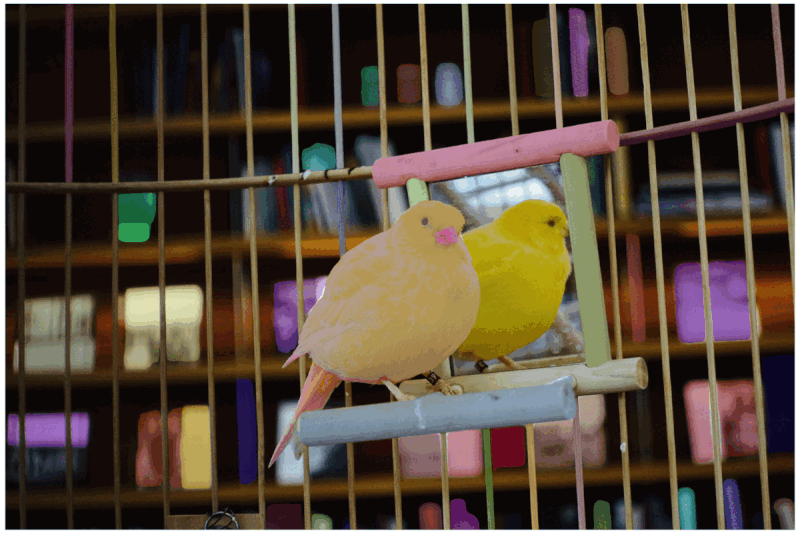SAM模型由三个部分组成。

提示编码器（Prompt Encoder ）：这是一个用于分割条件的编码器。可以使用以下条件进行分割提示：

1. 点（points）- 与应分割的对象相关的一组点。Prompt编码器使用位置编码将点转换为嵌入值。

2. 框（boxes）- 应分割的对象所在的边界框。类似于points，边界框的坐标通过位置编码来进行编码。

3. 分割掩码-由用户提供的分割掩码使用卷积进行嵌入，并与图像嵌入进行element-wise求和。

4. 文本（text）- 由CLIP模型编码的文本表示。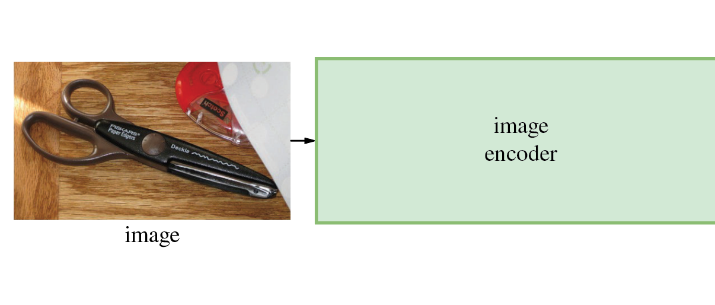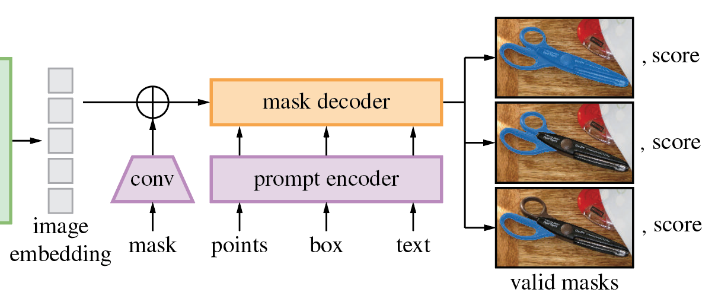https://github.com/openvinotoolkit/openvino_notebooks/tree/main/notebooks/237-segment-anything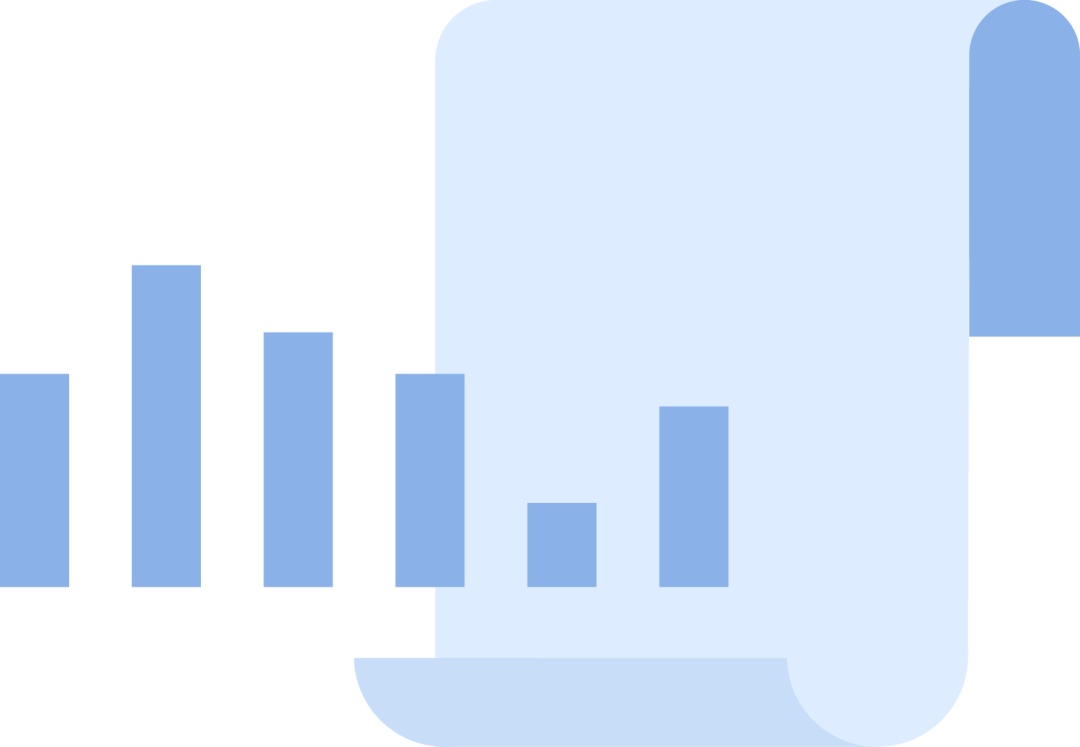!pip install -q "segment_anything" "gradio>=3.25"

  import sys    sys.path.append("../utils")  from notebook_utils import download_file    checkpoint = "sam_vit_b_01ec64.pth"  model_url = "https://dl.fbaipublicfiles.com/segment_anything/sam_vit_b_01ec64.pth"  model_type = "vit_b"    download_file(model_url)

  from segment_anything import sam_model_registry    sam = sam_model_registry[model_type](checkpoint=checkpoint)import warnings  from pathlib import Path  import torch  from openvino.tools import mo  from openvino.runtime import serialize, Core    core = Core()    ov_encoder_path = Path("sam_image_encoder.xml")    if not ov_encoder_path.exists():      onnx_encoder_path = ov_encoder_path.with_suffix(".onnx")      if not onnx_encoder_path.exists():          with warnings.catch_warnings():              warnings.filterwarnings("ignore", category=torch.jit.TracerWarning)              warnings.filterwarnings("ignore", category=UserWarning)                            torch.onnx.export(sam.image_encoder, torch.zeros(1,3,1024,1024), onnx_encoder_path)            ov_encoder_model = mo.convert_model(onnx_encoder_path, compress_to_fp16=True)      serialize(ov_encoder_model, str(ov_encoder_path))  else:      ov_encoder_model = core.read_model(ov_encoder_path)  ov_encoder = core.compile_model(ov_encoder_model)

image_embeddings：从image_encoder中嵌入的图像。具有长度为1的批索引。

point_coords：稀疏输入提示的坐标，对应于点输入和框输入。方框使用两个点进行编码，一个用于左上角，另一个用于右下角。坐标必须已转换为长边1024。具有长度为1的批索引。

point_labels：稀疏输入提示的标签。0是负输入点，1是正输入点，2是左上角，3是右下角，-1是填充点。*如果没有框输入，则应连接标签为-1且坐标为（0.0，0.0）的单个填充点。

iou_predictions-并集预测上的交集。import numpy as np  import cv2  import matplotlib.pyplot as plt    download_file("https://raw.githubusercontent.com/facebookresearch/segment-anything/main/notebooks/images/truck.jpg")  image = cv2.imread('truck.jpg')  image = cv2.cvtColor(image, cv2.COLOR_BGR2RGB)

  plt.figure(figsize=(10,10))  plt.imshow(image)  plt.axis('off')  plt.show()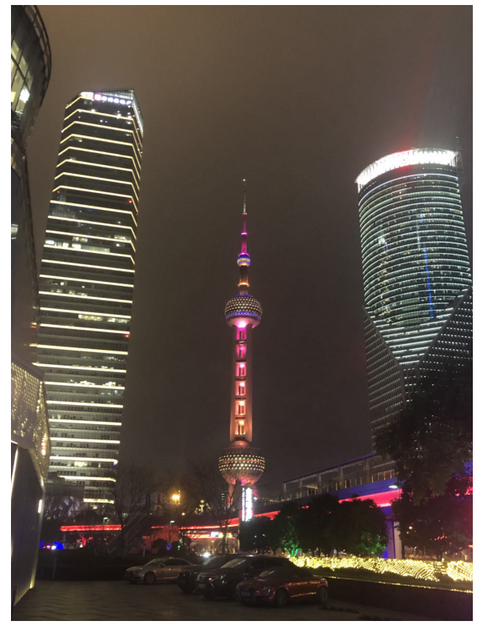将BGR图像转换为RGB

调整图像保存纵横比，其中最长尺寸等于图像编码器输入尺寸1024。

归一化图像减去平均值（123.675、116.28、103.53）并除以标准差（58.395、57.12、57.375）

将HWC数据布局转换为CHW并添加批次维度。

根据图像编码器预期的输入形状，按高度或宽度（取决于纵横比）向输入张量添加零填充。

  preprocessed_image = preprocess_image(image)  encoding_results = ov_encoder(preprocessed_image)    image_embeddings = encoding_results[ov_encoder.output(0)]

  input_point = np.array([[500, 375]])  input_label = np.array()    plt.figure(figsize=(10,10))  plt.imshow(image)  show_points(input_point, input_label, plt.gca())  plt.axis('off')  plt.show()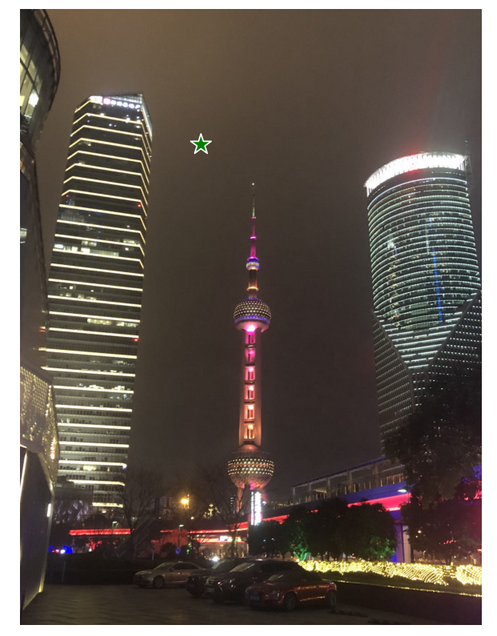coord = np.concatenate([input_point, np.array([[0.0, 0.0]])], axis=0)[None, :, :]  label = np.concatenate([input_label, np.array([-1])], axis=0)[None, :].astype(np.float32)  coord = resizer.apply_coords(coord, image.shape[:2]).astype(np.float32)

  inputs = {      "image_embeddings": image_embeddings,      "point_coords": coord,      "point_labels": label,  }

  results = ov_predictor(inputs)    masks = results[ov_predictor.output(0)]  masks = postprocess_masks(masks, image.shape[:-1])  masks = masks > 0.0

  plt.figure(figsize=(10,10))  plt.imshow(image)  show_mask(masks, plt.gca())  show_points(input_point, input_label, plt.gca())  plt.axis('off')  plt.show()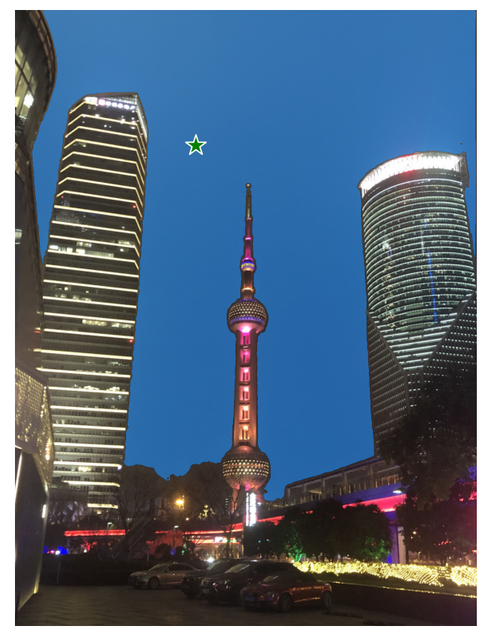input_point = np.array([[500, 375], [1125, 625], [575, 750])  input_label = np.array([1, 1, 1])

  plt.figure(figsize=(10,10))  plt.imshow(image)  show_points(input_point, input_label, plt.gca())  plt.axis('off')  plt.show()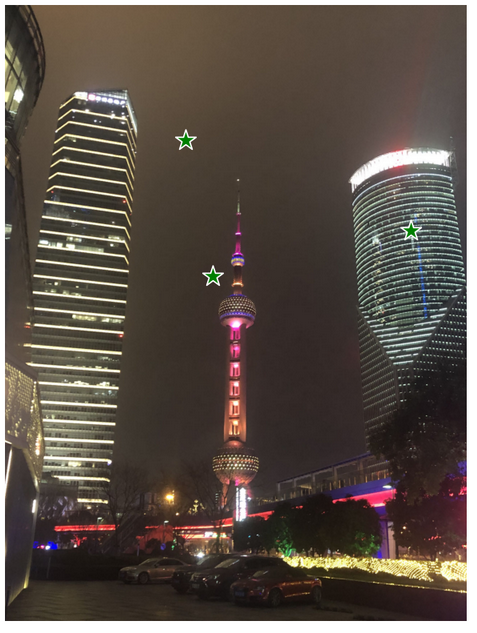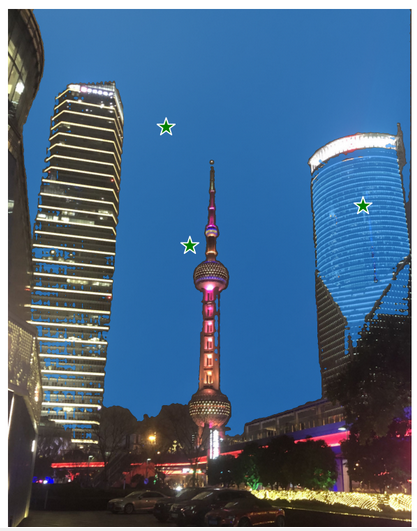input_box = np.array([425, 600, 700, 875])  input_point = np.array([[575, 750]])  input_label = np.array()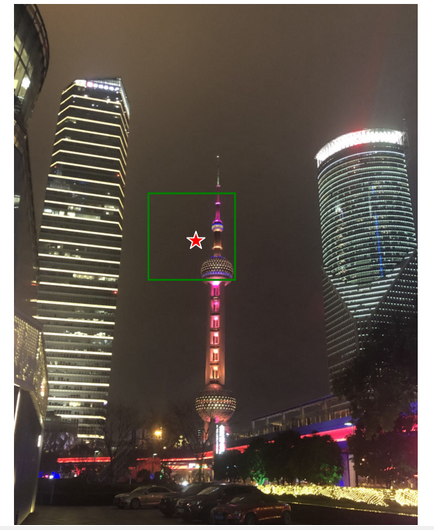box_coords = input_box.reshape(2, 2)  box_labels = np.array([2,3])    coord = np.concatenate([input_point, box_coords], axis=0)[None, :, :]  label = np.concatenate([input_label, box_labels], axis=0)[None, :].astype(np.float32)    coord = resizer.apply_coords(coord, image.shape[:2]).astype(np.float32)

  inputs = {      "image_embeddings": image_embeddings,      "point_coords": coord,      "point_labels": label,  }    results = ov_predictor(inputs)    masks = results[ov_predictor.output(0)]  masks = postprocess_masks(masks, image.shape[:-1])  masks = masks > 0.0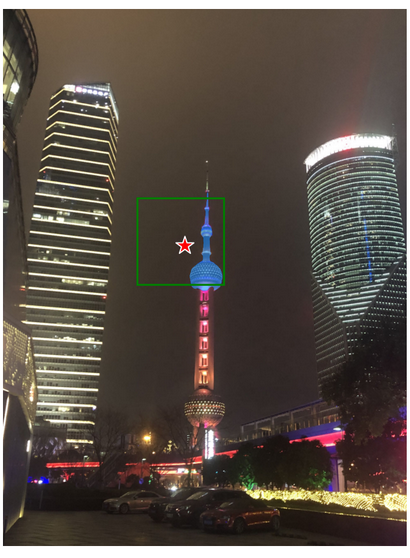from segment_anything.utils.amg import (      MaskData,       generate_crop_boxes,       uncrop_boxes_xyxy,       uncrop_masks,       uncrop_points,       calculate_stability_score,       rle_to_mask,       batched_mask_to_box,       mask_to_rle_pytorch,       is_box_near_crop_edge,      batch_iterator,      remove_small_regions,      build_all_layer_point_grids,      box_xyxy_to_xywh,      area_from_rle)  from torchvision.ops.boxes import batched_nms, box_area  from typing import Tuple, List, Dict, Any

  def automatic_mask_generation(      image: np.ndarray, min_mask_region_area: int = 0, points_per_side: int = 32, crop_n_layers: int = 0, crop_n_points_downscale_factor: int = 1, crop_overlap_ratio: float = 512 / 1500, box_nms_thresh: float = 0.7, crop_nms_thresh: float = 0.7) -> List[Dict[str, Any]]:      """      Generates masks for the given image.            Arguments:        image (np.ndarray): The image to generate masks for, in HWC uint8 format.            Returns:         list(dict(str, any)): A list over records for masks. Each record is           a dict containing the following keys:             segmentation (dict(str, any) or np.ndarray): The mask. If               output_mode='binary_mask', is an array of shape HW. Otherwise,               is a dictionary containing the RLE.             bbox (list(float)): The box around the mask, in XYWH format.             area (int): The area in pixels of the mask.             predicted_iou (float): The model's own prediction of the mask's               quality. This is filtered by the pred_iou_thresh parameter.             point_coords (list(list(float))): The point coordinates input               to the model to generate this mask.             stability_score (float): A measure of the mask's quality. This               is filtered on using the stability_score_thresh parameter.             crop_box (list(float)): The crop of the image used to generate               the mask, given in XYWH format.      """      point_grids = build_all_layer_point_grids(          points_per_side,          crop_n_layers,          crop_n_points_downscale_factor,      )      mask_data = generate_masks(          image, point_grids, crop_n_layers, crop_overlap_ratio, crop_nms_thresh)            # Filter small disconnected regions and holes in masks      if min_mask_region_area > 0:          mask_data = postprocess_small_regions(              mask_data,              min_mask_region_area,              max(box_nms_thresh, crop_nms_thresh),          )            mask_data["segmentations"] = [          rle_to_mask(rle) for rle in mask_data["rles"]]            # Write mask records      curr_anns = []      for idx in range(len(mask_data["segmentations"])):          ann = {              "segmentation": mask_data["segmentations"][idx],              "area": area_from_rle(mask_data["rles"][idx]),              "bbox": box_xyxy_to_xywh(mask_data["boxes"][idx]).tolist(),              "predicted_iou": mask_data["iou_preds"][idx].item(),              "point_coords": [mask_data["points"][idx].tolist()],              "stability_score": mask_data["stability_score"][idx].item(),              "crop_box": box_xyxy_to_xywh(mask_data["crop_boxes"][idx]).tolist(),          }          curr_anns.append(ann)            return curr_anns

  prediction = automatic_mask_generation(image)

分割：掩码

面积：掩码的面积（以像素为单位）

bbox：XYWH格式的掩码的边界框

predicted_out：模型自己对掩模质量的预测

point_coords：生成此掩码的采样输入点

稳定性核心：衡量掩码质量的一个附加指标

crop_box：用于以XYWH格式生成此掩码的图像的裁剪

  print(f"Number of detected masks: {len(prediction)}")  print(f"Annotation keys: {prediction.keys()}")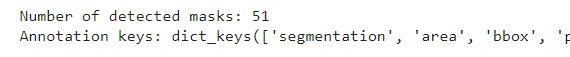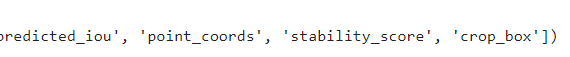from tqdm.notebook import tqdm    def draw_anns(image, anns):      if len(anns) == 0:          return      segments_image = image.copy()      sorted_anns = sorted(anns, key=(lambda x: x['area']), reverse=True)      for ann in tqdm(sorted_anns):          mask = ann["segmentation"]          mask_color = np.random.randint(0, 255, size=(1, 1, 3)).astype(np.uint8)          segments_image[mask] = mask_color      return cv2.addWeighted(image.astype(np.float32), 0.7, segments_image.astype(np.float32), 0.3, 0.0)

  import PIL    out = draw_anns(image, prediction)  cv2.imwrite("result.png", out[:, :, ::-1])    PIL.Image.open("result.png")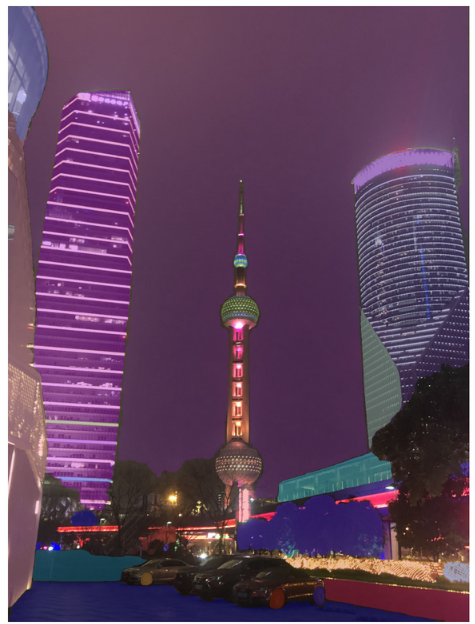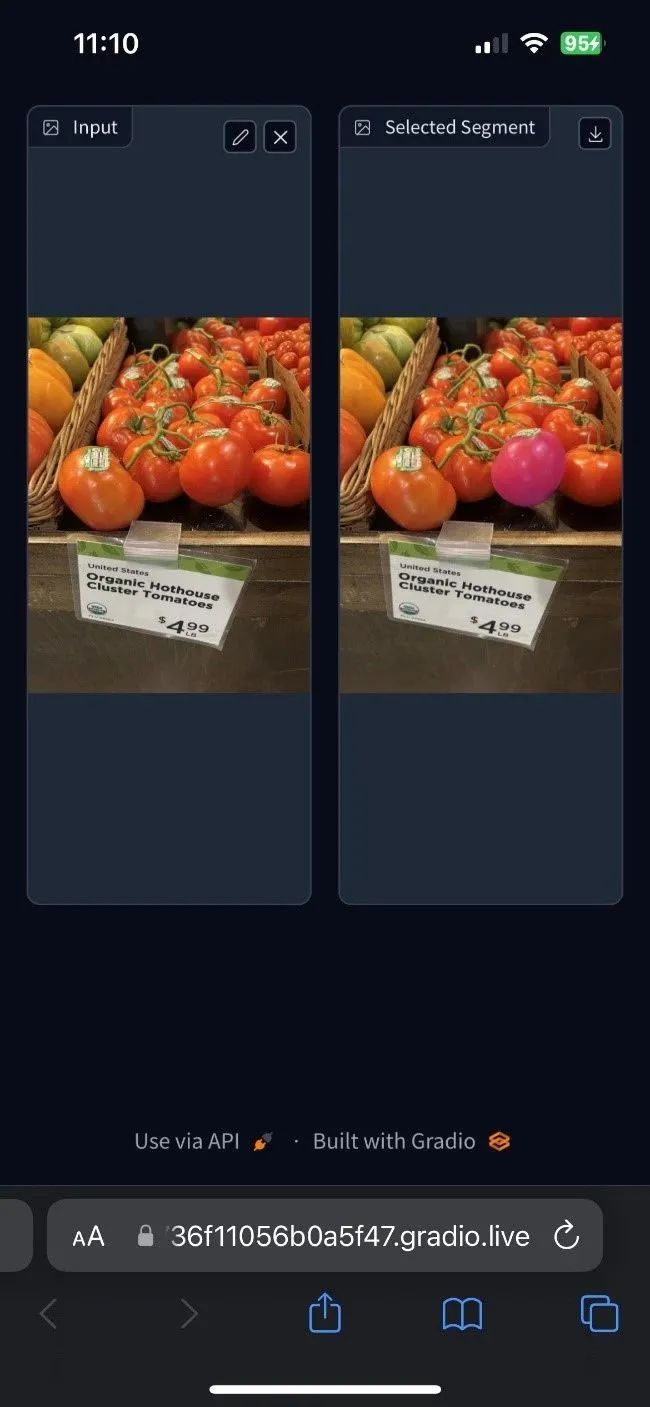https://github.com/openvinotoolkit/openvino_notebooks 。

--END--


你也许想了解(点击蓝字查看)⬇️

➡️ 开发者实战系列资源包来啦！

➡️

以AI作画，祝她节日快乐；简单三步，OpenVINO™ 助你轻松体验AIGC

➡️

还不知道如何用OpenVINO™作画？点击了解教程。

➡️

如何给开源项目做贡献? | 开发者节日福利

➡️

➡️

使用OpenVINO 在“端—边—云”快速实现高性能人工智能推理

➡️

图片提取文字很神奇？试试三步实现OCR！

➡️

【Notebook系列第六期】基于Pytorch预训练模型，实现语义分割任务

➡️

使用OpenVINO™ 预处理API进一步提升YOLOv5推理性能





扫描下方二维码立即体验
OpenVINO™ 工具套件 2022.3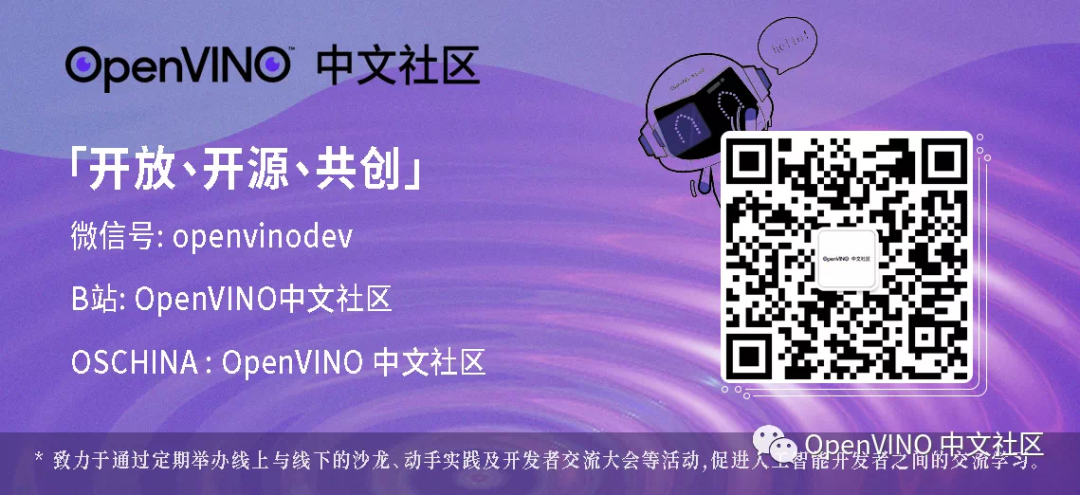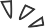0 评论
0 收藏
0